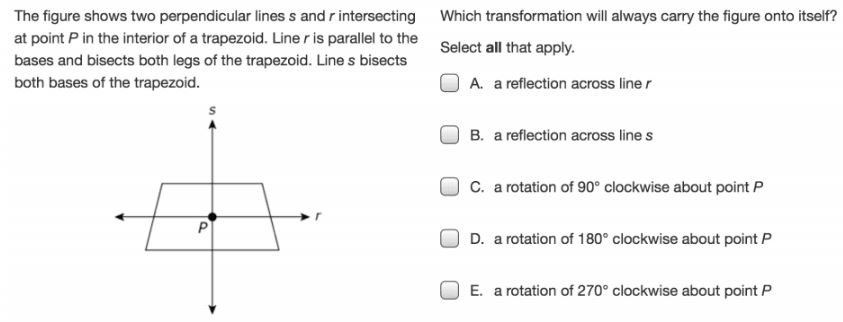1.2.3 Transformations Applications
starstarstarstarstarstarstarstarstarstar
by Jason Appel
| 6 Questions
Note from the author:
Playlist
The figure shown is a regular (all sides and angles congruent) octagon with its center at point C.1
1
10
Select each correct transformation.
A
B
C
D
E
F
Triangle ABC is graphed in the xy-coordinate plane, as shown.2
10
Triangle ABC (above) is reflected across the x-axis to form triangle A'B'C'. What are the coordinates of C' after the reflection?
(-6, 4)
(3, -2)
(4, -6)
(6, -4)3
3
10
Check all that apply
A
B
C
D
E
4
10
Triangle ABC has vertices at A(1,2), B(4,6) and C(4,2) in the coordinate plane. The triangle will be reflected over the x-axis and then rotated 180 degrees about the origin to form triangle A'B'C'. What are the vertices of triangle A'B'C'?
A'(1, -2), B'(4, -6), C'(4, -2)
A'(-1, -2), B'(-4, -6), C'(-4, -2)
A'(-1, 2), B'(-4, 6), C'(-4, 2)
A'(1, 2), B'(4, 6), C'(4, 2)5
5
10
Triangle ABC will be reflected across the line y=1 to form triangle A'B'C'.
Which quadrant(s) will contain at least one vertex of triangle A'B'C'? Check all that apply.
I
II
III
IV6
6
10
Select all that apply
A
B
C
D
E